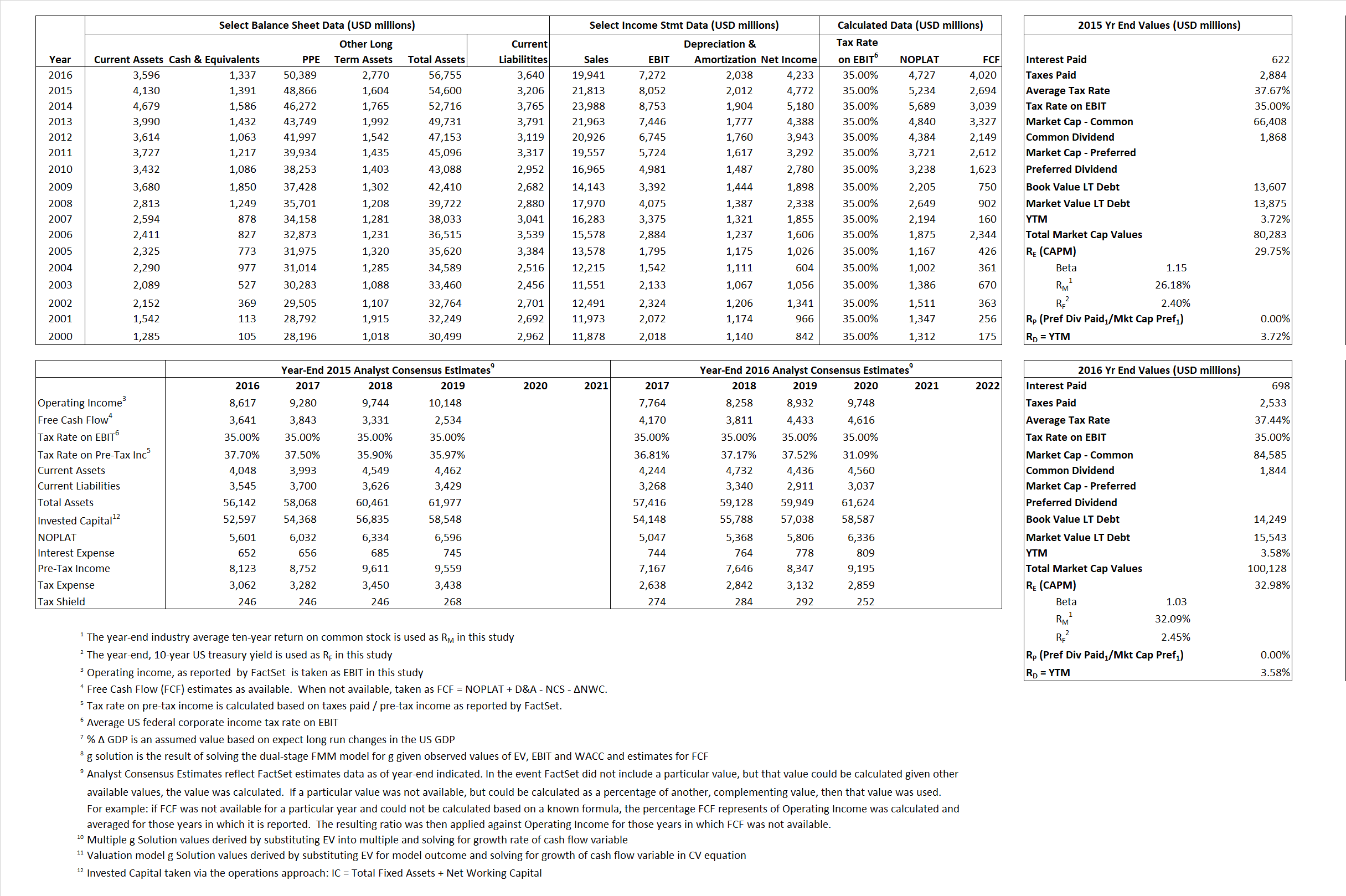# Union Pacific

## Analyst Listing

The following analysts provide coverage for the subject firm as of May 2016:

 Broker Analyst Analyst Email Credit Suisse Allison M. Landry allison.landry@credit-suisse.com Susquehanna Financial Group Bascome Majors bascome.majors@sig.com Cleveland Research Company Bryan Merolla bmerolla@cleveland-research.com TD Securities Cherilyn Radbourne cherilyn.radbourne@tdsecurities.com Bernstein Research David Vernon david.vernon@bernstein.com Avondale Partners Donald Broughton dbroughton@avondalepartnersllc.com BMO Capital Markets Fadi Chamoun fadi.chamoun@bmo.com Cowen & Company Jason H. Seidl jason.seidl@cowen.com Buckingham Research Jeffrey Kauffman jkauffman@buckresearch.com RBC Capital Markets John Barnes john.barnes@rbccm.com Argus Research John Eade jeade@argusresearch.com Stifel Nicolaus John G. Larkin jglarkin@stifel.com Stephens Inc Justin Long justin.long@stephens.com Morningstar Keith Schoonmaker keith.schoonmaker@morningstar.com BB&T Capital Markets Mark A. Levin mlevin@bbandtcm.com Nomura Research Matt Troy matt.troy@nomura.com Raymond James Patrick Tyler Brown tyler.brown@raymondjames.com Topeka Capital Markets Rick Paterson rp@topekacapitalmarkets.com Deutsche Bank Research Robert Salmon robert.salmon@db.com Wolfe Research Scott H. Group sgroup@wolferesearch.com Scotiabank GBM Turan Quettawala turan.quettawala@scotiabank.com

## Primary Input Data## Derived Input Data

### Equational Form

Net Operating Profit Less Adjusted Taxes NOPLAT  5,234  4,727$NOPLAT\, =\, EBIT\, x\, (1 \,-\, Avg \,\,Tax\,\, Rate\,\, on\,\, EBIT)$
Free Cash Flow FCF 2,694 4,020$FCF\,=NOPLAT\,+\,Non-Cash\,Expenses-\Delta NWC\,-\,NCS$
Tax Shield TS 234 261$TS\,=\,Interest\,\,Paid\,\,x\,\, Avg \,\,Tax\,\,Rate\,\, on\,\, Pre-Tax\,\, Income$
Invested Capital IC 51,394 53,115$IC\,=\,Fixed\,\,Operating\,\,Assets\,\,+\,\,Net\,\, Working\,\, Capital$
Return on Invested Capital ROIC 10.18% 8.90%$ROIC\,=\,\frac { NOPLAT }{ IC }$
Net Investment NetInv 4,455 3,759$NetInv\,=\,{ {IC}_{1}}-{{IC}_{0}}+Depreciation$
Investment Rate IR  85.12% 79.53%$IR\,=\,\frac {NetInv}{NOPLAT}$
Weighted Average Cost of Capital
WACCMarket 25.01% 28.21%$WACC\,=\,\frac { E }{ V } { R }_{ E }\,+\,\frac { P }{ V } { R }_{ P }\,+\,\frac { D }{ V } { R }_{ D }\left( 1- Avg\,\, Tax\,\,Rate\,\,on\,\,Pre-Tax\,\,Income \right)$
WACCBook  8.96%  8.05%
Enterprise value
EVMarket 78,892 98,791$EV\,=\,Market\,\,Cap\,\,Equity\,+\,\,Long\,\,Term\,\,Debt\,-\,Cash$
EVBook   78,678  97,497
Long-Run Growth
g = IR x ROIC
8.67%  7.08% Long-run growth rates of the income variable are used in the Continuing Value portion of the valuation models.
g = %$\Delta$ GDP    2.50%   2.50%
Margin from Operations M   36.91%  36.47%$M\,\,=\,\,\frac{EBIT}{SALES}$
Depreciation/Amortization Rate D  19.99%  21.89%$D\,\,=\,\,\frac{D+A}{EBITDA}$

## Valuation Multiple Outcomes

The outcomes presented in this study are the result of original input data, derived data, and synthesized inputs.

### model g solution

12/31/2015 12/31/2016 12/31/2015 12/31/2016 12/31/2015 12/31/2016

EV/SALES$\frac {EV}{Sales} \,= \,\frac{ROIC\, -\, g}{ROIC\,(WACC\,-\,g)}\,(1\,-\,T)\,(M)$

3.62  4.95 52.71%  50.66%  31.28% 33.00%

EV/EBITDA$\frac {EV}{EBITDA} \,= \,\frac{ROIC\, -\, g}{ROIC\,(WACC\,-\,g)}\,(1\,-\,T)\,(1\,-\,D)$

7.84 10.61 52.71% 50.66% 31.28% 33.00%

EV/NOPLAT$\frac {EV}{NOPLAT} \,= \,\frac{ROIC\, -\, g}{ROIC\,(WACC\,-\,g)}$

15.07 20.90 52.71% 50.66% 31.28% 33.00%

EV/FCFOPS$\frac {EV}{FCF_{OPS}} \,= \,\frac{ROIC\, -\, g}{ROIC\,(WACC\,-\,g)}\,(1\,-\,T)$

29.28 24.57 52.71% 50.66% 31.28% 33.00%

EV/EBIT$\frac {EV}{EBIT} \,= \,\frac{ROIC\, -\, g}{ROIC\,(WACC\,-\,g)}\,(1\,-\,T)$

9.80 13.59 52.71% 50.66% 31.28% 33.00%

EV/IC$\frac {EV}{IC} \,= \,\frac{ROIC\, -\, g}{WACC\,-\,g}$

1.54 1.86 52.71% 50.66% 31.28% 33.00%